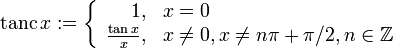# Tanc function

For functions involving angles (trigonometric functions, inverse trigonometric functions, etc.) we follow the convention that all angles are measured in radians. Thus, for instance, the angle of$90\,^\circ$ is measured as$\pi/2$.
The function is defined at all real numbers except odd multiples of$\pi/2$, and the definition is as follows:$\operatorname{tanc} \, x := \left\lbrace \begin{array}{rl} 1, & x = 0 \\ \frac{\tan x}{x}, & x \ne 0, x \ne n\pi + \pi/2, n \in \mathbb{Z} \end{array}\right.$